Next: Phenomenological Kick Velocity Up: Restrictions on the Scenario Previous: The COOC Criterion (Criterion

## Test of the Initial Mass-Ratio Distribution

Each run of our calculations consisted of computing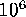evolutionary tracks for 10 equal intervals of the initial mass ratio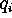=(0-0.1, 0.1-0.2, ..., 0.9-1.0) performed for seven values of the common envelope efficiency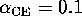, 0.3, 0.5, 1, 2, 3, 10 and 10 values of the kick velocity 0, 25, 50, 100, 200, 300, 400, 500, 700, 1000ĀkmĀs. In total, we obtained 700 matrices of stages. Then, for each value of the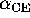and w we convolved the matrices from different intervals of mass ratio according to the trial initial mass ratio distribution for 50 values of the exponent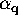ranging from 0 to 5 (that is, from a flat to a highly peaked toward unity initial distribution). Further, we convolved the matrices for the specified initial power law for the assumed kick velocity distribution (see the next section).

In our calculations we prohibited accretion-induced collapse (AIC) of Ā accreting WD, as otherwise we would get an excessive number of long-lived low-mass X-ray sources which would give an unobservably high X-ray luminosity of the Galaxy. However, we must note that the question of the AIC possibility for massive O-Ne-Mg WD deserves more detailed study. Here we only note that observational evidence favoring the AIC constantly appear in literature (e.g. Lipunov and Postnov (1985)).

The results of testingandparameters without kick velocity are presented in the form of "topographical maps" (i.e. as isolines) of constant ``COOC'' criterion in theplane calculated separately for each of the binary species listed in TableĀ4 (FiguresĀ16-20). The total COOC criterion calculated with equal weightsis shown in FigureĀ21.

ĀĀFigure 17: The same as shown in Figure 16 for the number of accreting NS with Be-stars.ĀĀ
Figure 16: The COOC criterion for the total X-ray luminosity of LMXB assuming no kick.Ā

ĀĀFigure 19: The same as shown in Figure 16 for the number of BH with massive OB-stars (Cyg X-1 like binaries).Ā
Figure 18: The same as shown in Figure 16 for the number of X-ray pulsars with OB-stars.Ā

ĀĀ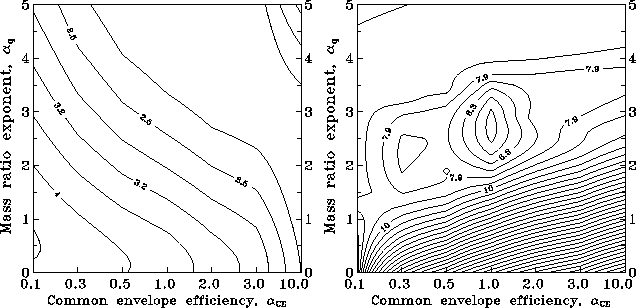Figure: The same as shown in Figure 16 for all the species from TableĀ4.Ā
Figure 20: The same as shown in Figure 16 for the number of cataclysmic variables.ĀĀ

It is clear from these figures that the best coincidence with the observations is reached when-3 and-10. A rather weak influence of the common envelopeĀ efficiency is connected with a wide log-normal distribution of binaries by initial semimajor axes, so only boundary effects (corresponding to the extreme values of) can change the results significantly.

As for the initial binary mass ratio distribution, it seems that at least for those binaries that produce NS during the course of their evolution, it must peak rather strongly toward unity, which is opposite to the widely used flat-like distributions (see, van den Heuvel, 1994); however, high mass ratios among massive unevolved binary O-stars appear to occur less frequently, thus confirming our conclusions (Garmany et al., 1980).

In FigureĀ22 we show how the total accretion X-ray luminosity of the LMXB depends on the parameterfor different values of the parameter. It is worth noting that forthe luminosity is essentially independent of the collapse anisotropyĀ and can be analytically fitted by the power law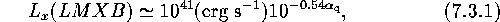for a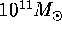spiralĀ galaxy with a constant star formation rate.

Ā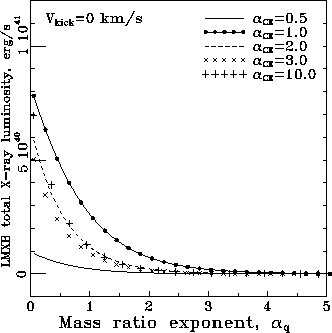Figure 22: Total X-ray luminosity of the galactic LMXB as a function offor different common envelope efficiencieswithout kick.ĀNext: Phenomenological Kick Velocity Up: Restrictions on the Scenario Previous: The COOC Criterion (Criterion

Mike E. Prokhorov
Sat Feb 22 18:38:13 MSK 1997